# beibehang Custom 3d mural wall paper TV backdrop sofa space to expand 3d photo wallpaper for walls 3 d flooring paper

(No reviews yet)
Condition:
New
Availability:
Imported
Options available
Shipping:
Calculated at Checkout
•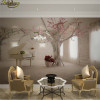•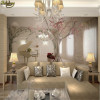•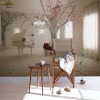•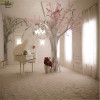•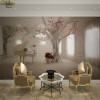•••••MSRP: ₹2,499
₹1,268
— You save ₹1,231
Frequently bought together:

## Description

• Charge Unit:Yuan/Roll
• Use:Elders' Room
• Use:Living Room
• Use:Kids' Room
• Use:Study
• Use:Bedding Room
• Use:Wedding House
• Function:Smoke-Proof
• Function:Fireproof
• Function:Waterproof
• Function:Sound-Absorbing
• Function:Soundproof
• Function:Anti-static
• Function:Mould-Proof
• Function:Heat Insulation
• Surface Treatment:Paper Wallpapers
• is_customized:Yes
• Feature:Mildew Resistant
• Feature:Extra Thick
• Feature:Environment Friendly
• Feature:Formaldehyde-free
• Usage:Entertainment
• Usage:Household
• Usage:Commerce
• Natural Material Wallpaper Material:PLANT
• Brand Name:beibehang
• Type:Natural Material Wallpapers
• Style:Europe
• Pattern:Tree
• Material:Wood fiber wallpaper
• Pattern:Personalized Customization

mural wallpaper price: \$ / square meter.    Not rolls.

1 square meter = 1pcs.   2 square meter=2pcs.   3 square meter =3pcs ...

Width x height = square meters = Order Quantity
Note: Please be careful to order 1m2. Because a very small size

We have 12 fixed size order.

Quantity: 1  = 1 s   quare meter        = 140cm (W) x 70cm (H) (4'3 "x 2'3") ft
Quantity: 2  = 2 s   quare meter        = 200cm (W) x 100cm (H) (6'5 "x 3'2") ft
Quantity: 3  = 3    s   quare meter        = 208cm (W) x 146cm (H) (6'8 "x 4'8") ft
Quantity: 4  = 4    s   quare meter        = 200cm (W) x 200cm (H) (6'5 '' x 6'5 '') ft
Quantity: 5  = 5     s   quare meter        = 250cm (W) x 200cm (H) (7'6 '' x6'5 '') ft
Quantity: 6 = 6     s   quare meter        = 300cm (W) x 200cm (H) (9'8 '' x 6'5 '') ft
Quantity: 7 =7    s   quare meter        = 300cm (W) x 250cm (H) (9'8 "x 8'2") ft
Quantity: 8 =8     s   quare meter        = 400cm (W) x 200cm (H) (13'6 "x 6'5") ft
Quantity: 9  =9      s   quare meter        = 350cm (W) x 260cm (H) (11'4 "x 8'7") ft
Quantity: 10 = 10     s   quare meter        = 360cm (W) x 280cm (H) (11'8 "x 9'2") ft
Quantity: 12 =12     s   quare meter        = 400cm (W) x 300cm (H) (13'6 "x 9'8") ft
Quantity: 16 =16     s   quare meter        = 500cm (W) x 320cm (H) (16'4 "x 10'5") ft

Other size . (width and height) . please tell us.

We will give you calculate the correct order quantity and price

FAQ: buyers ordered to leave. No size information. what to do?
We will wait for buyers 24 hours.
We will send         corresponding         default 12 fixed size.

FAQ:How to order other sizes?
Order other Size steps:
1: Select the correct quantity = square meter (width x height)
3: Fill in details .In the order number inside, write your size       information
4: Submit your order .Payments .on OK

Width x height = square meters (square feet)
1 square meter=10.76 square feet
tip:If the feet (inches) Give me information I will give you calculate the correct order quantity
For example        : You need to wall :Width = 2.8m  Height = 1.7m..
2.8m x 1.7m = 4.76m2 rounding .You need to order Quantity: 5

View AllClose

View AllClose